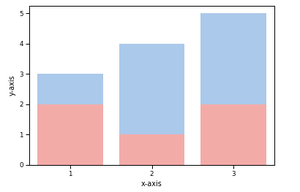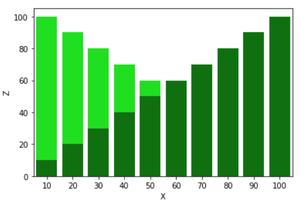GeeksforGeeks App
Open AppBrowser
Continue

# How to overlap two Barplots in Seaborn?

Seaborn is an amazing visualization library for statistical graphics plotting in Python. It provides beautiful default styles and colour palettes to make statistical plots more attractive. It is built on the top of matplotlib library and also closely integrated to the data structures from pandas.

Bar Plot is used to represent categories of data using rectangular bars. We can overlap two barplots in seaborn by creating subplots.

Steps required to overlap two barplots in seaborn:

1. Importing seaborn and matplotlib library,  seaborn for plotting graph and matplotlib for using subplot().
2. Creating dataframe.
3. Creating two subplots on the same axes.
4. Displaying the plot.

Below are some examples based on the above approach:

Example 1:

## Python3

 `# importing all required libraries``import` `pandas as pd``import` `seaborn as sns``import` `matplotlib.pyplot as plt` `# creating dataframe``df ``=` `pd.DataFrame({``    ``'X'``: [``1``, ``2``, ``3``],``    ``'Y'``: [``3``, ``4``, ``5``],``    ``'Z'``: [``2``, ``1``, ``2``]``})` `# creating subplots``ax ``=` `plt.subplots()` `# plotting columns``ax ``=` `sns.barplot(x``=``df[``"X"``], y``=``df[``"Y"``], color``=``'b'``)``ax ``=` `sns.barplot(x``=``df[``"X"``], y``=``df[``"Z"``], color``=``'r'``)` `# renaming the axes``ax.``set``(xlabel``=``"x-axis"``, ylabel``=``"y-axis"``)` `# visualizing illustration``plt.show()`

Output:Example 2:

## Python3

 `#importing all required libraries``import` `pandas as pd``import` `seaborn as sns``import` `matplotlib.pyplot as plt` `#creating dataframe``df``=``pd.DataFrame({``    ``'X'``:[i ``for` `i ``in` `range``(``10``,``110``,``10``)],``    ``'Y'``:[i ``for` `i ``in` `range``(``100``,``0``,``-``10``)],``    ``'Z'``:[i ``for` `i ``in` `range``(``10``,``110``,``10``)]``})` `#creating subplots``ax``=``plt.subplots()` `#plotting columns``ax``=``sns.barplot(x``=``df[``"X"``],y``=``df[``"Y"``],color ``=` `'lime'``)``ax``=``sns.barplot(x``=``df[``"X"``],y``=``df[``"Z"``],color ``=` `'green'``)` `#renaming the axes``ax.``set``(xlabel``=``"x-axis"``, ylabel``=``"y-axis"``)` `# visualizing illustration``plt.show()`

Output:My Personal Notes arrow_drop_up